#Function Repository Resource:

# MultiplicationTable

Generate a multiplication table for a range of integers, optionally using modular arithmetic

Contributed by: Paco Jain (Wolfram Research)
 ResourceFunction["MultiplicationTable"][n] produces the multiplication tables for positive integers less than n. ResourceFunction["MultiplicationTable"][{n, m}] produces the multiplication tables for positive integers between n and m.

## Details and Options

ResourceFunction["MultiplicationTable"] returns a Grid expression.
MultiplicationTable takes Modulus as an option, which specifies that arithmetic should be performed with the indicated modulus. The default value of 0 indicates ordinary arithmetic.

## Examples

### Basic Examples

Produce a basic multiplication table:

 In:=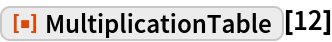Out=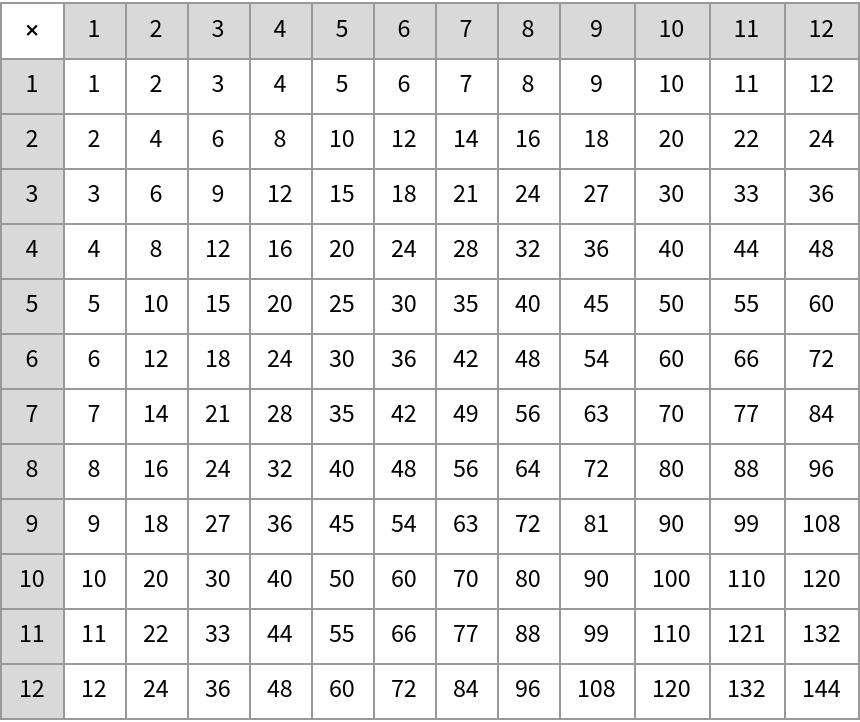Produce a multiplication table across a specific range of values:

 In:=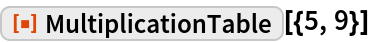Out=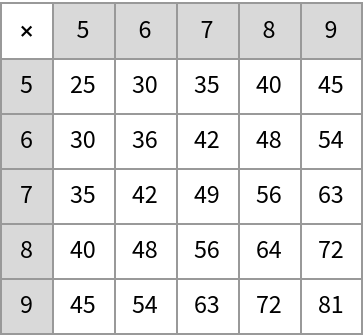### Options

Produce a multiplication table using modular arithmetic:

 In:=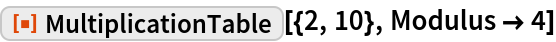Out=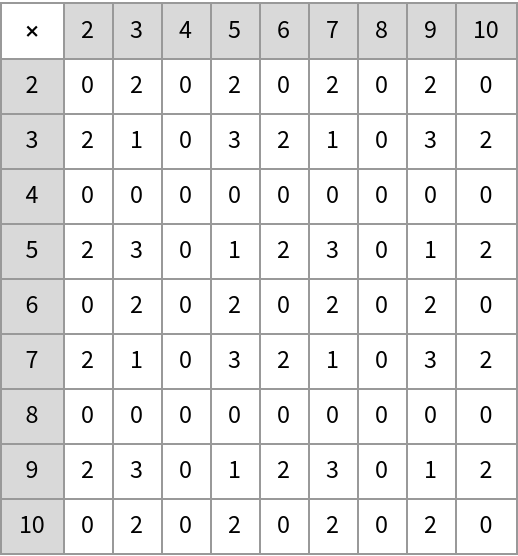## Requirements

Wolfram Language 11.3 (March 2018) or above